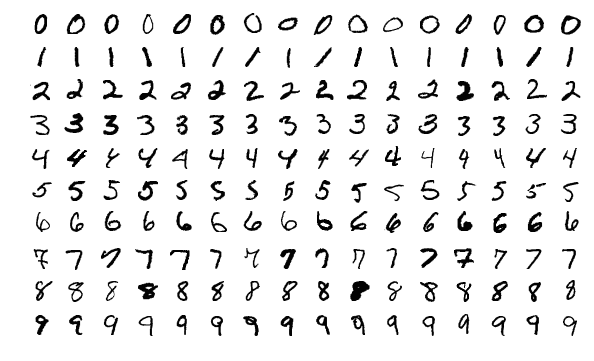What is PyTorch

PyTorch is a Machine Learning (ML) framework based on Torch. Torch is a Tensor library like Numpy, but unlike Numpy, Torch has strong GPU support.

You can use Torch either using the Lua programming language or if you favor Python like I do, you can use PyTorch.

No need to mention you may use PyTorch together with all major Python packages like scipy, Numpy, matplotlib and Cython and benefit with PyTorch’s autograd system.In here I assume you are eager to do some machine learning work, or you already tried PyTorch.

Although PyTorch did many great things, I found PyTorch website is missing some examples, especially how to load datasets.

This is why I am providing here the example of how to load the MNIST dataset.

In this example we use the PyTorch class DataLoader from torch.utils.data. This will download the resource from Yann Lecun’s website.

Yann Lecun is chief AI Scientist at Facebook, if he is still there as you are reading this.

MNIST dataset has 70.000 images in total where 60.000 images represent the train set and 10.000 images are the validation (test) set.

import torch
import torch.nn as nn
import torch.nn.functional as F
from torch.utils.data import DataLoader, Dataset, TensorDataset
from torch.optim import *
import torchvision
import torchvision.transforms as transforms
import matplotlib.pyplot as plt
import os
import numpy as np
import random

dev = torch.device("cuda") if torch.cuda.is_available() else torch.device("cpu")
bs=512

t = transforms.Compose([
transforms.ToTensor(),
transforms.Normalize(mean=(0), std=(1))]
)

batch_size=bs, drop_last=True, shuffle=True)
batch_size=bs, drop_last=True, shuffle=True)

Create the model

Once you have the data loaders you need the model. Here we will use a model with the ResBlock inside.

class ResBlock(nn.Module):
def __init__(self, nf):
super().__init__()

self.conv1 = nn.Conv2d(nf, nf, kernel_size=3, stride=1, padding=1, bias=False)
self.bn1 = nn.BatchNorm2d(nf)
self.relu = nn.ReLU(inplace=True)
self.conv2 = nn.Conv2d(nf, nf, kernel_size=3, stride=1, padding=1, bias=False)
self.bn2 = nn.BatchNorm2d(nf)

def forward(self, x):
o = self.conv1(x)
o = self.bn1(o)
o = self.relu(o)
o = self.conv2(o)
o = self.bn2(o)
o = o + x
o = self.relu(o)
return o

# kernel of 3x3, stride of 2 and padding 1
def convk3s2p1(ni, nf):
return nn.Sequential(
nn.Conv2d(ni, nf, 3, 2, 1, bias=False),
nn.BatchNorm2d(nf),
nn.ReLU()
)

model=nn.Sequential(
convk3s2p1(1, 8),
ResBlock(8),
convk3s2p1(8, 16),
ResBlock(16),
convk3s2p1(16, 32),
ResBlock(32),
convk3s2p1(32, 16),
convk3s2p1(16, 10),
nn.Flatten()
).to('cuda')

# read 85 as BS (batch size)
input=(torch.randn(85, 1, 24, 24))
output=model(input.to('cuda'))
print(output.shape)
model

The optimizer and the loss function

Once you have the model you need the optimizer and the loss function.

optimizer = torch.optim.SGD(model.parameters(), lr=0.5, momentum=0.9)
loss_fn = nn.CrossEntropyLoss()

Once you have all that you need a train loop.

epochs=10
losses=[]

total_steps=len(dl_train)*epochs
scheduler = torch.optim.lr_scheduler.OneCycleLR(optimizer,
max_lr=0.5,
steps_per_epoch=len(dl_train),
epochs=epochs)

from sklearn.metrics import accuracy_score, f1_score

for epoch in range(1,epochs+1):
print(f"Epoch {epoch}")

# TRAINING PHASE
model.train()

ect=0 # epoch correct test
ecv=0 # epoch correct validation
ett=0 # len of epoch train examples
etv=0 # len of epoch validation examples

for i, (input,target) in enumerate(dl_train):

if(i%10==0): print(i, end=" ")

input = input.to(dev)
target = target.to(dev)

output = model(input)
ect+= accuracy_score(output.argmax(dim=-1).cpu(), target.cpu(), normalize=False)

loss = loss_fn(output, target) # one batch loss
losses.append(loss.item())
loss.backward()
optimizer.step()
scheduler.step()
ett+=len(target)

# VALIDATION PHASE
model.eval()
for j, (input,target) in enumerate(dl_valid):

input = input.to(dev)
target = target.to(dev)
output = model(input)
ecv+= accuracy_score(output.argmax(dim=-1).cpu(), target.cpu(), normalize=False)
etv+=len(target)

print("")
print("Epoch training accuracy" , ect/ ett)
# experiment.log_metric("Epoch training accuracy", ect/ ett)
print("Epoch valid accuracy" , ecv/ etv)
# experiment.log_metric("Epoch valid accuracy", ecv/ etv)

The results:

Epoch 1
0 10 20 30 40 50 60 70 80 90 100 110
Epoch training accuracy 0.9074519230769231
Epoch valid accuracy 0.968030427631579
Epoch 2
0 10 20 30 40 50 60 70 80 90 100 110
Epoch training accuracy 0.9788828792735043
Epoch valid accuracy 0.9822162828947368
Epoch 3
0 10 20 30 40 50 60 70 80 90 100 110
Epoch training accuracy 0.9845753205128205
Epoch valid accuracy 0.9815995065789473
Epoch 4
0 10 20 30 40 50 60 70 80 90 100 110
Epoch training accuracy 0.9887319711538461
Epoch valid accuracy 0.987047697368421
Epoch 5
0 10 20 30 40 50 60 70 80 90 100 110
Epoch training accuracy 0.9914863782051282
Epoch valid accuracy 0.9903371710526315
Epoch 6
0 10 20 30 40 50 60 70 80 90 100 110
Epoch training accuracy 0.9930889423076923
Epoch valid accuracy 0.9899259868421053
Epoch 7
0 10 20 30 40 50 60 70 80 90 100 110
Epoch training accuracy 0.9957098023504274
Epoch valid accuracy 0.9922902960526315
Epoch 8
0 10 20 30 40 50 60 70 80 90 100 110
Epoch training accuracy 0.9979801014957265
Epoch valid accuracy 0.9924958881578947
Epoch 9
0 10 20 30 40 50 60 70 80 90 100 110
Epoch training accuracy 0.9990985576923077
Epoch valid accuracy 0.9923930921052632
Epoch 10
0 10 20 30 40 50 60 70 80 90 100 110
Epoch training accuracy 0.9995826655982906
Epoch valid accuracy 0.9923930921052632

Reproducing the experiment

To reproduce the exactly same results I used this function and set the seed to 12.

def set_seed(seed):
torch.manual_seed(seed)
torch.cuda.manual_seed_all(seed)
torch.backends.cudnn.deterministic = True
torch.backends.cudnn.benchmark = False
np.random.seed(seed)
random.seed(seed)

tags: tricks - mnist - dataset - manual - loading - pytorch - reproducing & category: pytorch